Journal of Electrical and Electronic Engineering
Volume 4, Issue 6, December 2016, Pages: 150-156

Feasibility Study on Intelligent Evaluation of Marine Traffic Congestion Degree for Restricted Water Using Fuzzy Expert System with AIS Report

Emmanuel Nartey*, Isaac Owusu-Nyarko

Department of Electrical/Electronic, Regional Maritime University, Accra, Ghana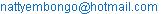(E. Nartey)

*Corresponding author

Emmanuel Nartey, Isaac Owusu-Nyarko. Feasibility Study on Intelligent Evaluation of Marine Traffic Congestion Degree for Restricted Water Using Fuzzy Expert System with AIS Report. Journal of Electrical and Electronic Engineering. Vol. 4, No. 6, 2016, pp. 150-156. doi: 10.11648/j.jeee.20160406.12

Received: November 22, 2016; Accepted: November 30, 2016; Published: January 3, 2017

Abstract: In this paper we investigate the modification of feasibility study on Intelligent Evaluation Marine Traffic Congestion Degree for Restricted Water Area which has now become one of the numerous challenging factors to improve the safety of navigation. However, the observation of marine traffic using Fuzzy Expert with the aid of AIS acquired knowledge from the collected data enable the congestion degree to be visualize and possible to estimate Restricted Water Area base on traffic congestion degree with main flow velocity. The formation, aggregation, and decomposition of rules explained fuzzy mathematical tools and the calculus of IF-THEN rules provides a most useful paradigm for the automation and implementation of an extensive body of human knowledge. Therefore, the clarification includes a discussion of fuzzification and defuzzification strategies, the definition of fuzzy implication and an analysis of fuzzy reasoning mechanism. Now IMO is trying to standardize criterion of Universal AIS. As you know AIS is now studied and has been studied in many advanced country as a tools to exchange information in both ship-to-ship and ship-to-shore in congested waterways. The intention of standardization is to improve safety of VTS management and to support effective navigation of ships.

Keywords: Marine Traffic, Expect Systems, Fuzzy Logic, Fuzzy Sets, AIS

1. Introduction

The quest to make life activities less cumbersome and easier cuts across every ports and channels due to the traffic congestion as a result, the rate of developing vessel and its advancement is increasing swiftly in order to achieve the world growing economic demands.

The standardization criterion of Universal AIS is now obligation for vessels to carry AIS Class A which entered into force in July 2008 and with the fitted AIS receiver into the radar network system as full implementation by IMO. In general, AIS is now useful tool and its characteristics have been studied in many advanced country as a tools to exchange information in both ship-to-ship and ship-to-shore in congested waterways. The intention of standardization is to improve safety of VIS management and to support effective navigation of ships. For example, avoiding of collision, tools of VTS, sending cargo information to ships and to local center, automatic flow of ship's information, estimation of evasive area etc. Figure 1 shows the concept of Universal AIS. Vessel fitted with AIS sends message in one time slot and at the same it reserves another time slot for next position message.

All other AIS-equipped ships repeat with position reports of all vessels in restricted waters, evaluation of traffic congestion degree could be achieved. However, in restricted waters, there may be only partial vessels equipped with AIS while others may not comply according to the regulations.

A fuzzy expects system is characterized by a set of linguistic statements based on expert knowledge. The expert knowledge can be express in the form of IF-THEN rules, which are easily implemented by fuzzy conditional statements in fuzzy logic.

In this paper we focus on the following

(1)  Fuzzy expert system to determine traffic congestion degree with main flow velocity.

(2)  Evaluation of the main traffic flow velocity with speed information provided by AIS reports.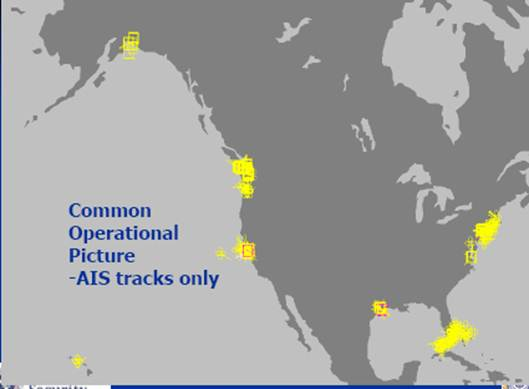Figure 1. Shows the concept of Universal AIS.

2. Literature Review and Theoretical Framework

2.1. Fuzzy Inference System (Fuzzy Expert System)

Fuzzy inference is the process of formulating the mapping from a given input to an output using fuzzy logic. The mapping then provides a basis from which decisions can be made, or patterns discerned. Fuzzy inference systems (FISs) are also known as fuzzy rule-based systems, fuzzy model, fuzzy expert system, and fuzzy associative memory. This is a major unit of a fuzzy logic system. According to Bellman, R. E., Zadeh, L. A. (1970), decision-making is an important part in the traffic congestion system. The FIS formulates suitable rules and based upon the rules the decision is made. This is mainly based on the concepts of the fuzzy set theory, fuzzy IF–THEN rules, and fuzzy reasoning. FIS uses "IF...THEN..." statements, and the connectors present in the rule statement are "OR" or "AND" to make the necessary decision rules. The basic FIS can take either fuzzy inputs or crisp inputs, but the outputs it produces are almost always fuzzy sets. When the FIS is used as a controller, it is necessary to have a crisp output. Therefore in this case defuzzification method is adopted to best extract a crisp value that best represents a fuzzy set. The whole FIS is discussed in detail in the following subsections.

Fuzzy inference systems have been successfully applied in fields such as automatic control, data classification, decision analysis, expert systems, and computer vision. Because of its multidisciplinary nature, fuzzy inference systems are associated with a number of names, such as fuzzy-rule-based systems, fuzzy expert systems, fuzzy modeling, fuzzy associative memory, fuzzy logic controllers, and simply (and ambiguously) fuzzy systems.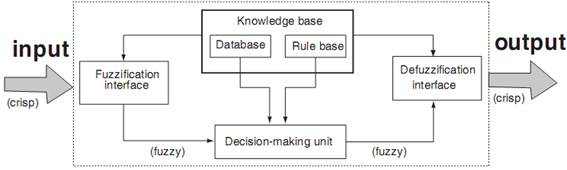Figure 2. Fuzzy Inference System.

Mandeni’s fuzzy inference method is the most commonly seen fuzzy methodology. Mandeni’s method was among the first control systems built using fuzzy set theory. It was proposed in 1975 by Ebrahim Mandeni as an attempt to control a steam engine and boiler combination by synthesizing a set of linguistic control rules obtained from experienced human operators. Mandeni’s effort was based on Lotfi Zadeh’s 1973 paper on fuzzy algorithms for complex systems and decision processes.

2.2. Construction and Working of Inference System

Fuzzy inference system consists of a fuzzification interface, a rule base, a database, a decision-making unit, and finally a defuzzification interface. A FIS with five functional block described in Figure 1. The function of each block is as follows:

a rule base containing a number of fuzzy IF–THEN rules;

a database which defines the membership functions of the fuzzy sets used in the fuzzy rules;

a decision-making unit which performs the inference operations on the rules;

a fuzzification interface which transforms the crisp inputs into degrees of match with linguistic values; and

a defuzzification interface which transforms the fuzzy results of the inference into a crisp output.

The working of FIS is as follows. The crisp input is converted in to fuzzy by using fuzzification method. After fuzzification the rule base is formed. The rule base and the database are jointly referred to as the knowledge base. Defuzzification is used to convert fuzzy value to the real world value which is the output.

The working of FIS is as follows. The crisp input is converted in to fuzzy by using fuzzification method. After fuzzification the rule base is formed. The rule base and the database are jointly referred to as the knowledge base. Defuzzification is used to convert fuzzy value to the real world value which is the output.

The steps of fuzzy reasoning (inference operations upon fuzzy IF–THEN rules) performed by FISs are:

Compare the input variables with the membership functions on the antecedent part to obtain the membership values of each linguistic label. (This step is often called fuzzification.)

Combine (through a specific t-norm operator, usually multiplication or min) the membership values on the premise part to get firing strength (weight) of each rule.

Generate the qualified consequents (either fuzzy or crisp) or each rule depending on the firing strength.

Aggregate the qualified consequents to produce a crisp output. (This step is called defuzzification.)

2.3. Fuzzy Inference Methods

The most important two types of fuzzy inference method are Mandeni’s fuzzy inference method, which is the most commonly seen inference method. This method was introduced by Mandeni and Assilian (1975). Another well-known inference method is the so-called Sugeno or Takagi–Sugeno–Kang method of fuzzy inference process. This method was introduced by Sugeno (1985). This method is also called as TS method. The main difference between the two methods lies in the consequent of fuzzy rules. Mandeni fuzzy systems use fuzzy sets as rule consequent whereas TS fuzzy systems employ linear functions of input variables as rule consequent. All the existing results on fuzzy systems as universal approximators deal with Mandeni fuzzy systems only and no result is available for TS fuzzy systems with linear rule consequent.

2.4. Mamdani’s Fuzzy Inference Method

Mandeni’s fuzzy inference method is the most commonly seen fuzzy methodology. Mandeni’s method was among the first control systems built using fuzzy set theory. It was proposed by Mandeni (1975) as an attempt to control a steam engine and boiler combination by synthesizing a set of linguistic control rules obtained from experienced human operators. Mandeni’s effort was based on Zadeh’s (1973) paper on fuzzy algorithms for complex systems and decision processes.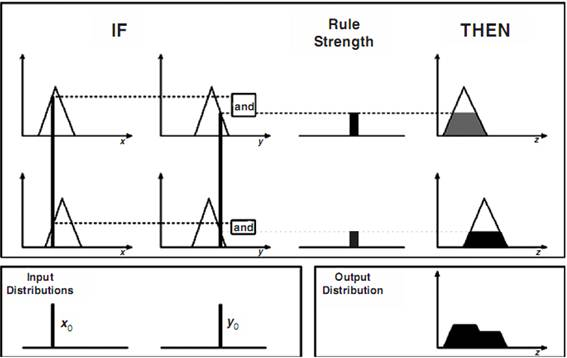Figure 3. A Two Inputs, Two Rules Mandeni FIS with Crisp Inputs.

Mamdani type inference, as defined it for the Fuzzy Logic Toolbox, expects the output membership functions to be fuzzy sets. After the aggregation process, there is a fuzzy set for each output variable that needs defuzzification. According to Chang, S. S. L., Zadeh, L. A. (1972), it is possible, and in many cases much more efficient, to use a single spike as the output membership functions rather than a distributed fuzzy set. This is sometimes known as a singleton output membership function, and it can be thought of as a pre-defuzzified fuzzy set. It enhances the efficiency of the defuzzification process because it greatly simplifies the computation required by the more general Mandeni method, which finds the centroid of a two dimensional functions. Rather than integrating across the two-dimensional function to find the centroid, the weighted average of a few data points. Sugeno type systems support this type of model. In general, Sugeno type systems can be used to model any inference system in which the output membership functions are either linear or constant. An example of a Mandeni inference system is shown in figure 3. To compute the output of this FIS given the inputs, six steps have to be followed:

1)    Determining a set of fuzzy rules

2)    Fuzzifying the inputs using the input membership functions

3)    Combining the fuzzified inputs according to the fuzzy rules to establish a rule strength

4)    Finding the consequence of the rule by combining the rule strength and the output membership function

5)    Combining the consequences to get an output distribution

6)    Defuzzifying the output distribution (this step is only if a crisp output (class) is needed).

3. System Construction

To construct the fuzzy inference system, we select one input and one output.

We make Ship Velocity as input and Traffic Congestion as output.

We set,

Input = Ship Velocity

Output = Traffic Congestion

We have to determine degree of membership for the Ship Velocity and Traffic Congestion, we construct the fuzzy set, and therefore we set

Ship Velocity= {Slow, Normal, Fast}

Traffic Congestion= {Smooth, Normal, Block}

We construct the Fuzzy Rule Using the Fuzzy Inference Systems (FISs)

First Rule: If the velocity is fast, then Traffic Congestion is smooth

Second Rule: If the velocity is normal, then Traffic Congestion is normal

Third Rule: If the velocity is slow, then Traffic Congestion is block.

Table 1. Membership of Ship Velocity and Traffic Congestion.

 Grade Membership for Ship Velocity Membership for Traffic Congestion 1 Slow Block 2 Normal Normal 3 Fast Smooth

Figure 4: shows the construction of the fuzzy inference System and table 1 shows the membership of ship velocity and traffic congestion.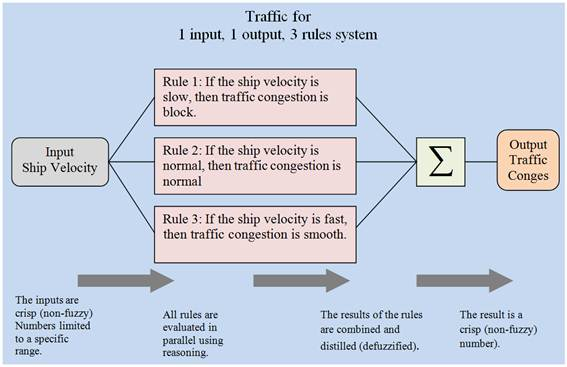Figure 4. The Construction of The Fuzzy Inference System.

4. Result and Discussion

We use the Madman’s fuzzy inference method for traffic congestion and also with the aid of matlab (R2010b) to run the fuzzy inference system. To construct the degree of membership of input and output, we select the range of input and output from the collected data with the aid of AIS report.

We set, Input range=[0 24] and Output range=[0 24]

The results can be seen in the following figures and table. Figure 5, 6, 7, 8, 9, 10 and 11 illustrating the system traffic congestion, degree membership of ship velocity, degree membership of traffic congestion, surface of ship velocity and traffic congestion, ruler view viewer for rule 1, 2 and 3.

Table 2 shows the results of output (traffic congestion) for input (ship velocity) according to the rules. When ship velocity is 4, traffic congestion is 20. We found out that, when the ship velocity is slow, traffic congestion is block. When we take the ship velocity is 12, traffic congestion is 12. This show, when the ship velocity is normal, traffic congestion is normal. When the ship velocity is 20, traffic congestion is 4. We can see that, when the ship velocity is fast, the traffic congestion is smooth. The outfit of this result can be analyzed from the Mamdani’s inference method which is useful for traffic congestion in restricted water areas. Advantages of the Mamdani’s Method are as follows;

It is intuitive,

It is well suited to human input.

Fuzzy inference system is the most important modeling tool based on fuzzy set theory. The FISs are built by domain experts and are used in automatic control, decision analysis, and various other experts systems.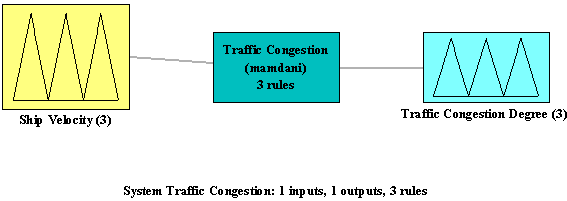Figure 5. System Traffic Congestion.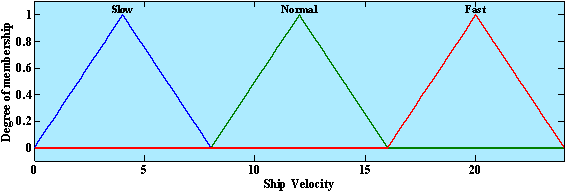Figure 6. Degree Membership of Ship Velocity.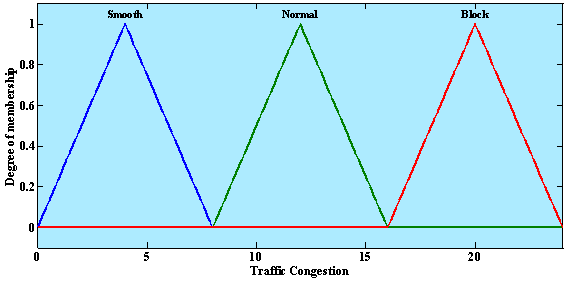Figure 7. Degree Membership of Traffic Congestion.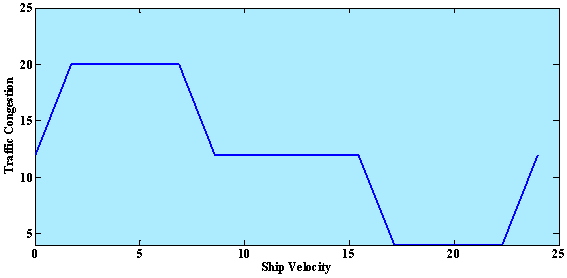Figure 8. Surface of Ship Velocity and Traffic Congestion.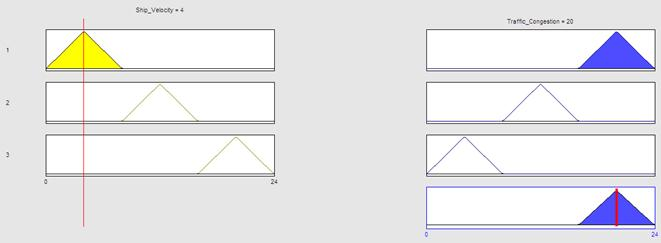Figure 9. Rule Viewr of Rule 1.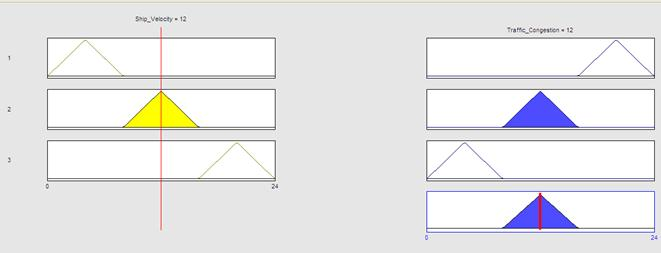Figure 10. Rule Viewr of Rule 2.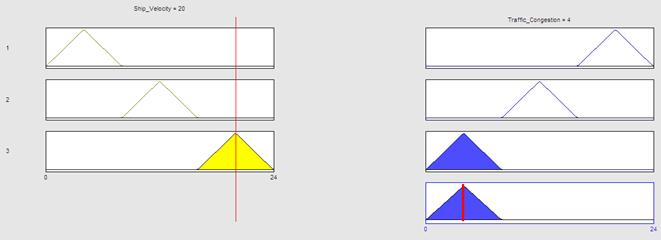Figure 11. Rule Viewer of Rule 3.

Table 2. The Results of Input and Output.

 Rules Input (Ship Velocity) Output (Traffic Congestion) 1 4 20 2 12 12 3 20 4

5. Conclusion

The fuzzy logic Toolbox in Matlab can be used for various applications. The membership values are assigned easily. The application developed is capable of executing within a fraction of second. The fuzzification and the defuzzification process are performed using command line functions as. The user can obtain the output in any form.

It is well know that systems control encounters difficulties in satisfying multiple performance indices simultaneously and in achieving accurate control in the presence of disturbances. I n such circumstances, fuzzy expert provide an effective framework for solution. Perhaps, the state evaluation fuzzy control does not evaluate the computed control actions as human operators do. By contrast, the predictive fuzzy expert control system provides a mechanism for evaluation so that the desired states and control objectives can be achieved more easily.

The aim of this study is to investigate and modify the evaluation of marine traffic congestion degree using fuzzy to estimate Restricted Water Area base on traffic congestion degree with main flow velocity to improve the safety of navigation.

References

1. Deepa, S. N., Savanandam, S. N., and Sumathis, S. (2007). Introduction to fuzzy logic using MATLAB.
2. The MathWorks Inc., (1995-2010).Fuzzy Logic Toolbox™ User’s Guide.
3. Lopez de Mantaras, R., Agusti, J. Plaza, E., Sierra, C. (1991).A fuzzy expert system shell. In: Kandel A (Ed) Fuzzy expert systems. CRC, Boca Raton, FL.
4. Zadeh, L. A. (1973). The calculus of fuzzy if–then rules.
5. Yasunobu, S., Myamoto, S. (1985). Automatic train operation by predictive fuzzy control. Industrial applications of fuzzy control. North-Holland, Amsterdam.
6. Dubois, D., Prade, H. (1990). An introduction to possibility and fuzzy logics. In: Shafer G, Pearl J (Eds) Readings in uncertain reasoning. Morgan Kaufmann, San Francisco.
7. Dubois, D., Prade, H. (1998). Soft computing, fuzzy logic, and artiﬁcial intelligence, soft computing a fusion of foundations, methodologies and applications.
8. Bauer, P., Bodenhofer, U., Klement, E. P. (1996). A fuzzy algorithm for pixel classiﬁcation based on the discrepancy norm. In: Proceedings of 5th IEEE 8.international conference on fuzzy systems, New Orleans, LA, September vol 3, pp. 2007–2012.
9. Zadeh, L. A.(1965). Fuzzy set. Inform. Contr., vol. 8, pp. 338-353.
10. Chang, S. S. L., Zadeh, L. A.(1972).Fuzzy mapping and control. IEEE Trans. Syst., Man, Cybern., vol.SMC-2, pp. 30-34.
11. Bellman, R. E., Zadeh, L. A.(1970). Decision-making in a fuzzy environment. Magement Sci., vol. 17, pp. B-141-B-164.

 Contents 1. 2. 2.1. 2.2. 2.3. 2.4. 3. 4. 5.
Article ToolsAbstractPDF(442K)